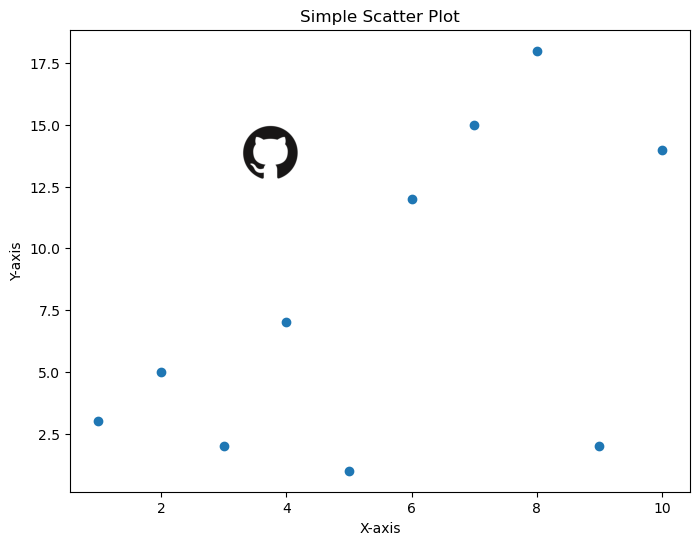## Introduction

Adding a logo to your matplotlib graphics can be a bit tricky when you've never done it before. With this in mind, let's take a look at what to do if you want to add an image or logo to your beautiful matplotlib graphics.

## Libraries

First, you need to install the following librairies:

• matplotlib for displaying the chart and the logo
• `numpy` is used for manipulating the image as an array
• `PIL` for opening the image
• `requests` is used to send an HTTP GET request to the specified URL to download the image content
• `io` is used to create a file-like object in memory to hold the image content that is downloaded using the requests library

Don't forget to install PIL if you haven't already done so with the `pip install Pillow` command.

``````# Libraries
from PIL import Image
import requests
from io import BytesIO
import numpy as np
import matplotlib.pyplot as plt``````

## Open or Load the image

We'll look at two cases: using an image stored locally on your computer and using an image from the Internet using only the url address.

• Image stored locally: make sure you write the right path (in the following example, `/Users/josephbarbier/Desktop/python graph gallery/github_logo.png` is the location of the image on my computer). The image I chosed is just a basic png github logo.
• Image from the Internet: we need to make sure we complete the objective with some test. With the `if` statement, we are checking whether the HTTP response status code is equal to 200. In the context of HTTP, a status code of 200 means "OK", indicating that the request was successful and the server has responded with the requested content. If this test is passed, then we just have to get the content from the request and open the image!
``````# Open an image from a computer
def open_image_local(path_to_image):
image = Image.open(path_to_image) # Open the image
image_array = np.array(image) # Convert to a numpy array
return image_array # Output

# Open the image from my computer
image = open_image_local('/Users/josephbarbier/Desktop/python graph gallery/github_logo.png')``````
``````# Open an image from the Internet

# Make sure everything went right
if response.status_code == 200:
image_bytes = BytesIO(response.content) # Get content
image = Image.open(image_bytes) # Open the image
return image # Output
else:

# Open image from the Internet
image = open_image_internet('https://github.githubassets.com/images/modules/logos_page/GitHub-Mark.png')``````

## Create a simple plot with a logo

Now let's create a simple graph with a logo at bottom left. First, we have to define some data and plot them. In our case, we will create a scatter plot since it's a very simple chart.

Once we've done that, we need to define some parameters:

• `x-axis position`
• `y-axis position`
• `Image width`
• `Image height`

It's difficult to set these automatically, as the appropriate values may vary from one graph to another. Unless you have something else, the simplest thing to do is to test several positions and dimensions until you find the best one.

``````# Some data
x = [1, 2, 3, 4, 5, 6, 7, 8, 9, 10]
y = [3, 5, 2, 7, 1, 12, 15, 18, 2, 14]

# Create scatter plot
fig, ax = plt.subplots(figsize=(8, 6))

# Scatter plot
ax.scatter(x, y)

ax.set_xlabel('X-axis')
ax.set_ylabel('Y-axis')
ax.set_title('Simple Scatter Plot')

# Define the position and size parameters
image_xaxis = 0.3
image_yaxis = 0.6
image_width = 0.15
image_height = 0.15  # Same as width since our logo is a square

# Define the position for the image axes
image_yaxis,
image_width,
image_height]
)

# Display the image
ax_image.imshow(image)
ax_image.axis('off')  # Remove axis of the image

# Display the plot
plt.show()``````